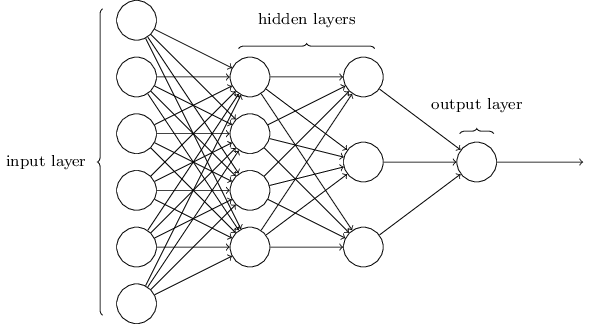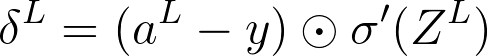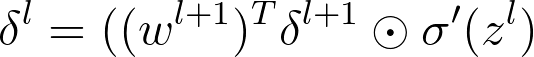Simon Maxwell-Stewart
September 18 3 min

# Making a neural network from scratch using Go

After beginning a long journey to educate myself in machine learning (ML), and more recently artificial intelligence (AI), I started hearing the same piece of advice over and over again…

You need to try implementing your own neural network from scratch.

As a developer I have trained and inferred from many “out the box” models, and even made my own with the help of high-level frameworks, but I have never implemented my own simple neural network without the use of any frameworks. I was feeling a little daunted to slog my way through the hours of linear algebra. Thankfully I work with some truly awesome scientists, and one of them was looking to learn Go (aka Golang).

We decided to implement the simplest of neural network (NN) a multi-layer-perceptron (MLP). While being relatively simple, MLPs are still complex things to fully grasp, and so provide a really excellent learning opportunity to understand the core principles of AI.Multi-Layered Perceptron (MLP) from Neural Networks and Deep Learning.

For the data we will use the famous Iris dataset from the UC Irvine Machine Learning Repository. This is a small dataset, but nicely demonstrates the ability of NN’s to accurately predict a label for a data point, based on it’s input features.

NOTE — We used gonum / mat for the matrix linear algebra maths stuff, because WHY WOULD YOU EVER BUILD YOUR OWN LINEAR ALGEBRA LIBRARY!

### The theory

In this article I won’t be going in to the theory of NNs, as I could never do justice to the excellent resources that already exist out there. Out of all of them we both thought chapter 1 and 2 of Neural Networks and Deep Learning, as an excellent explanation of the processes we need to implement in our code.

We will use this book as reference, so if you need a refresher in basic NN theory I suggest you read the first two chapters now. The code below may be cryptic to most developers without this knowledge. And if you aren’t used to looking at linear algebra… start, like seriously, it’s ok to just look at it. Get a feel for it.

### The interface

So let’s think about what a NN should look like at a high level. We should be able to train it with labelled data so it can learn a model to describe the data we feed it. Also we need to evaluate it’s performance, as we are building a classifier, we will use accuracy as a suitable metric. Finally when we know our net is ready to fly into the unkown, we want it to predict on a given dataset.

If we were to write this in Go, it would look something like this…

### The Guts

At it’s core a feed-forward NN represents two processes, a forward pass — receiving input data and returning activations from the net, and a backward pass — receiving ground truth and updating the net’s weights and biases.

forward()

According to Neural Networks and Deep Learning the forward pass consists of computing the activation, σ(wx+) at each layer, where >em class="markup--em markup--p-em">σ(z)=1/1+ez and x is the input from the previous layer. In the below function we return both the ‘z’s and the activations as we will need them for the backward pass.

As you can see this is pretty straight forward to implement. So far so good!

backward()

So the backward pass is a lot trickier to comprehend intuitively. To understand this properly you must take time to really understand the back-propagation algorithm and the motivations behind it’s creation. The big idea here is that we are calculating the gradient of our error (how far away the predictions are from ground truth) with respect to our weights and biases at every layer and then updating our weights and biases by changing their value in the direction of least error.

First we need to compute the“delta” of the last layer, here L denotes the last layer…Then we need to use this result to calculate the “delta” of the previous layers, working backwards, here l denotes the lth layer…Once we have our deltas at each layer it is simple to calculate the gradients of the error for the biases and weights…Finally once we have these gradients we can update our weights and biases by performing gradient descent, e.g. updating values in opposite direction of the gradient. Here we are showing the formula for performing this update for a “batch” of inputs, so we take the average by summing the gradients and dividing it by the amount of inputs in our batch…Ok so translating all of this into code we end up with something like below. Be warned understanding how these equations are implemented as various matrix operations is the central difficulty of this exercise. It takes time to get it!

### The Wrapping

So now we have the implementation done for the core functionality we can wrap these functions up to satisfy the interface we require…

#### train()

To train we simply feed batches of our training data set, x and y, into our backward pass, and loop for a number of epochs; the amount of times we want to train over our dataset.

#### predict()

Predict is very simple to implement, we simply want the final activation from a forward pass.

#### evaluate()

To evaluate the network, we give it some test data, x, and the corresponding ground truth y. We generate predictions from x and the calculate the accuracy by counting the amount of times it predicted the correct class according to our ground truth.

### The Conclusion

After finishing this exercise I totally get why it is so recommended. Completing it, you understand the fundamental processes of NNs in much more detail, such as back-propagation, and how matrix operations are used to implement these algorithms. It is easy to think you understand these ideas because you “get it” at a high-level, but implementing your own NN leaves you nothing to hide behind.

That being said, here are some tips to get the most out of this exercise…

• Don’t simply copy paste my code or anyone else’s. Look, but write your own!
• Do it your way. While looking at other people’s code you may be confused as to why they did it a certain way, don’t worry about it too much, if in doubt, try your own implementation according to your own intuitions.
• Have a friend who is good at linear algebra who you can bug with incessant questions. Thank you Parmida. ;)